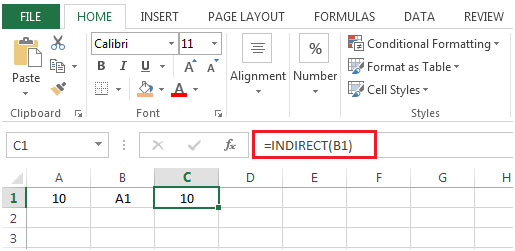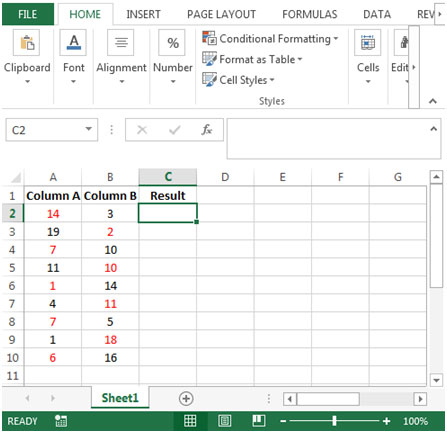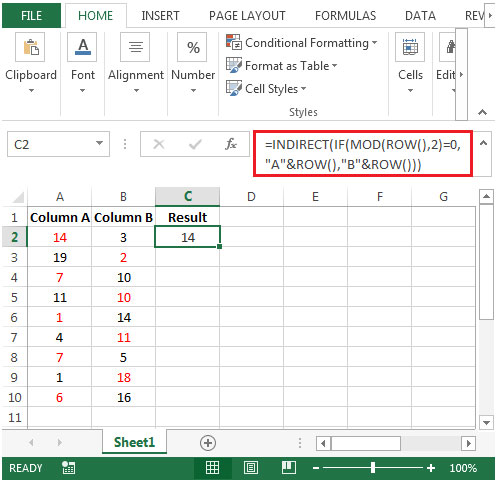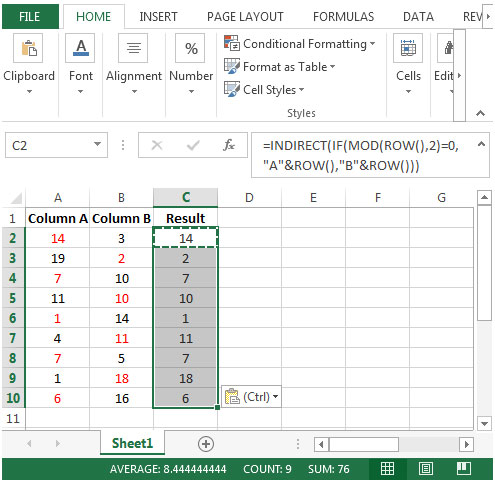# Retrieving Each Second Number from Two Lists

To retrieve each second number from two lists, we will use combination of IF, MOD, ROW & INDIRECT functions to get the output.

The IF function checks if a condition you specify is TRUE or FALSE. If returns TRUE then returns preset value, if returns FALSE then returns another preset value.

Syntax = IF(logical_test,value_if_true,value_if_false)

logical_test: logical test will test the condition or criteria, if condition meets then return the preset value, if condition not meet then return the other preset value.

value_if_true: The value that you want to be returned if this argument returns TRUE.

value_if_false: The value that you want to be returned if this argument returns FALSE

MOD: Returns the remainder after a number is divided by a divisor.

Syntax =MOD(number,divisor)

number: It is a numeric value whose remainder you want to find.

divisor: It is the number which is used to divide the number argument.

ROW: Returns the row number of a reference.

Syntax: =ROW(reference)

Reference: It is a reference to a cell or range of cells.

INDIRECT: Returns the reference specified by a text string.

Syntax: =INDIRECT(ref_text,A1)

ref_text is a reference to a cell. If ref_text is not a valid cell reference, then INDIRECT function will return #REF error.

The second argument A1 refers to a logical value that specifies type of reference is contained in the cell ref_text.

If a1 is TRUE or omitted then ref_text is determined as A1-style reference.

If a1 is FALSE then ref_text is determined as R1C1 style reference.

Example:If value in cell A1 contains 10, B1 contains A1& we use INDIRECT function in cell C1=INDIRECT(B1), then result would be 10Let us take an example:

We have some random numbers in column A & B.We need a formula to retrieve each second number from two lists. The required number is colored in red.• In cell C2, the formula would be
• =INDIRECT(IF(MOD(ROW(),2)=0,"A"&ROW(),"B"&ROW()))
• Press enter on your keyboard.• By copying down the formula from cell C2 to range C3:C10, we will get the desired result.You will get the every second number in sequence from two list.

Terms and Conditions of use

The applications/code on this site are distributed as is and without warranties or liability. In no event shall the owner of the copyrights, or the authors of the applications/code be liable for any loss of profit, any problems or any damage resulting from the use or evaluation of the applications/code.